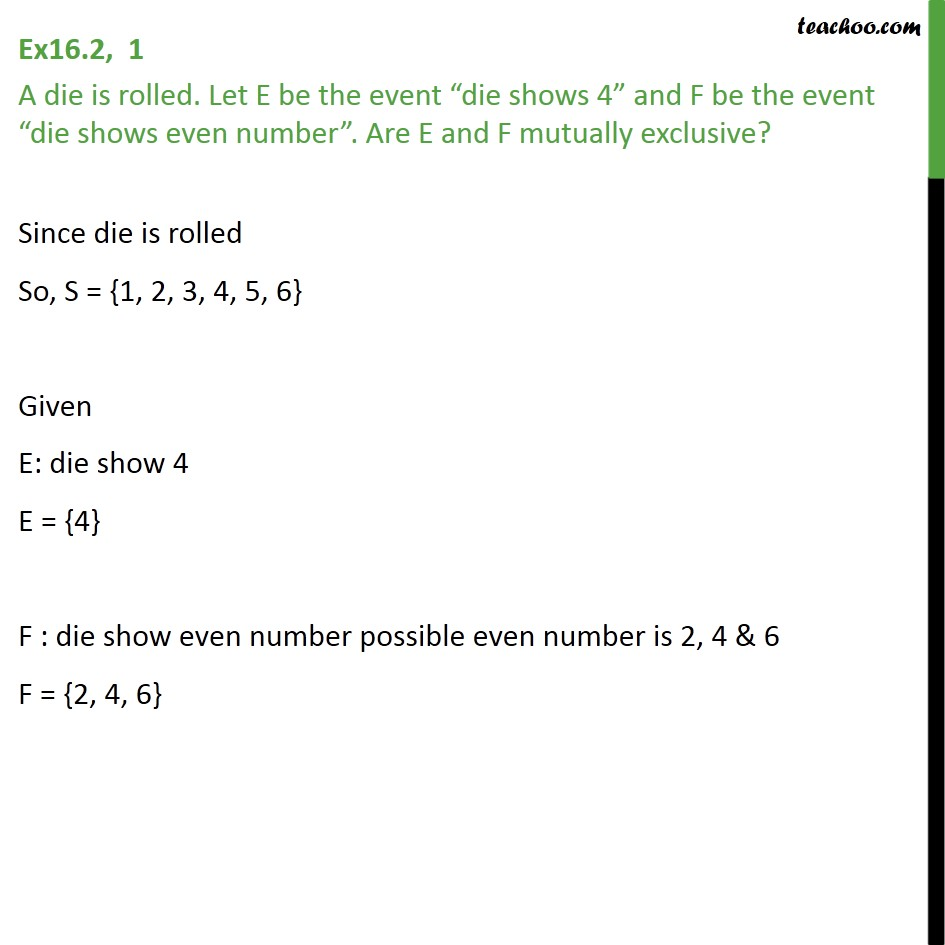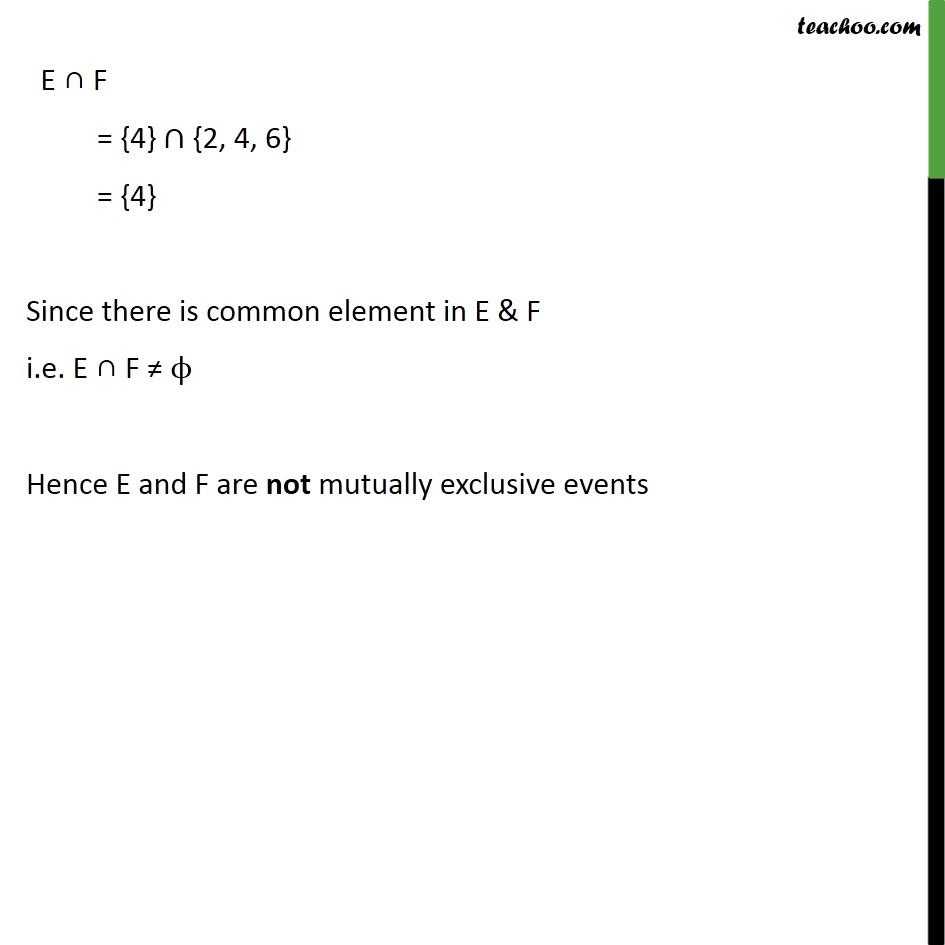Ex 16.2

Chapter 16 Class 11 Probability (Term 2)
Serial order wise### Transcript

Ex16.2, 1 A die is rolled. Let E be the event die shows 4 and F be the event die shows even number . Are E and F mutually exclusive? Since die is rolled So, S = {1, 2, 3, 4, 5, 6} Given E: die show 4 E = {4} F : die show even number possible even number is 2, 4 & 6 F = {2, 4, 6} E F = {4} {2, 4, 6} = {4} Since there is common element in E & F i.e. E F Hence E and F are not mutually exclusive events